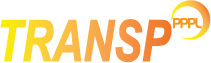# Fusion products

TRANSP can follow the fusion products using the NUBEAM MonteCarlo.NPTCLF
Number of particles to be tracked in the MonteCarlo code. The default is 1000
NLFST
Set equal to TRUE to calculate products from D(D,T)p reactions [Eq.1].
NLFSP
Set equal to TRUE to follow protons from D(D,T)p reactions [Eq.1].
NLFHE3
Set equal to TRUE to calculate products from D(D,He3)n reactions [Eq.2].
NLFHE4
Set equal to TRUE to calculate alpha products from D(T,He4)n reactions {Eq.3}.
NLFATOM
Set equal to TRUE to compute atomic physics effects on fusion products fast ions slowing down.

The T(T,He4)2n reactions [Eq.4], as a by-product of D(D,T)p reactions, are also calculated in TRANSP.

### New fitting for T-T reactivities

With version 18.2 new fittings over DPC have been implemented for T(T,2n)4He fusion reactions, based on the work by David Coster [IPP, Garching].
The input data are available on the SVN repository

Set:

LEV_TTNUK = 1
To use the new fittings by David Coster. The figure below shows a comparison between the old fitting in TRANSP and the new fit by D. Coster.

## Power threshold

It is possible to set a power threshold above which the number of particles NPTCLF in the MonteCarlo calculations is maintained. If the actual source power is lower than the set power threshold, then a population of f*NPTCLF MonteCarlo particles is maintained, where f=[actual source power]/threshold power. The default values for the various fusion reactions are as follow:

PLFST [=100.0 W]
power threshold for D(D,T)p reactions
PLFSP [=100.0 W]
power threshold for D(D,T)p reactions
PLFHE3 [=100.0 W]
power threshold for D(D,He3)n reactions
PLFHE4 [=10.0 kW]
power threshold for D(T,He4)n reactions

## Model Options for alphas

NALPHA=0
This is the default value, to calculate the alphas from the MonteCarlo model.
NALPHA=1
Fast, non-Monte Carlo model by D. Mikkelsen. This model has no orbit effects and assumes instant equilibration of the alpha slowing down distribution on the background plasma.

## Renormalisation

It is possible to use 1D input Ufile data for the total production rates of the various fast ion populations as a function of time to renormalise the profiles determined from simulation. This is done using the following switches:

NLUSFA
Set this flag to `TRUE` to read in He4 data from UFILES `PRESFA/EXTSFA`.
NLUSF3
Set this flag to `TRUE` to read in He3 data from UFILES `PRESF3/EXTSF3`.
NLUSFT
Set this flag to `TRUE` to read in He3 data from UFILES `PRESFT/EXTSFT`.
NLUSFP
Set this flag to `TRUE` to read in He3 data from UFILES `PRESFP/EXTSFP`.

## MonteCarlo model approximations

Included:
• orbit effects, orbit loss, thermalization source.
• collisional heating of the background plasma.
• time dependent evolution of the slowing down population.
• nuclear burnup of D-D Tritons or He3.
• atomic physics effects of/on the fusion product ions (e.g. charge exchange loss of fusion product fast ions) [activated with NLFATOM=.T.]
• accumulation of slowed down "ash" thermal ions in the background plasma (if the appropriate species is included).
Limitation:
• fusion ion source function is isotropic and monoenergetic in the plasma reference frame.
Not included:
• collisional coupling between fast ion populations of different species